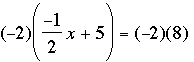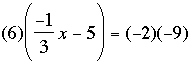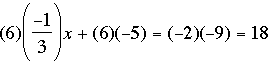SEARCH HOMEMath Central Quandaries & QueriesQuestion from Michelle, a student: How would I solve: (-2)(-1/2x + 5) = (-2)(8)?Hi Mitchelle,

By the way you have written the equation I'm not sure if the x is in the numerator or denominator. I am going to assume your equation is. I'm going to show you haw to solve this by looking at a similar problem.

Solve.

On the right side -2 times -9 is 18. On the right I would multiply through by 6 using the distributive law. Thus I get.

Six times minus a third is minus two and six times minus five is minus thirty so the equation can be written

-2x - 30 = 18.

Multiply both sides of the equation by -1 to get

(-1)(-2x - 30) = (-1)(18)

or

2x + 30 = -18.

Adding -30 to each side produces

2x = -18 - 30 = -48

and thus x = -24.

Now try your equation,
PennyMath Central is supported by the University of Regina and The Pacific Institute for the Mathematical Sciences.